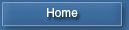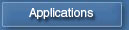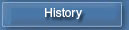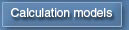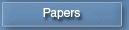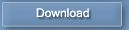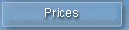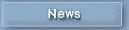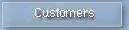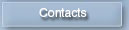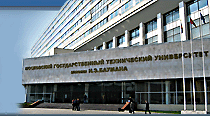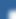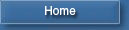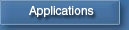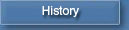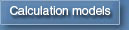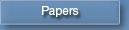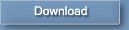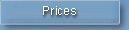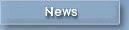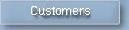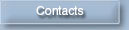Diesel-RK in Telegram## Simulation of Nitrogen Oxides formation in cylinder of ICE

Dr., Professor Vasiliy Zvonov,    Dr. Andrey Kozlov

At simulation of nitrogen oxides formation it is accepted that the cylinder is divided into two zones: zone of fresh charge and zone of burnt gas. Zone of fresh charge consists of air, fuel and residual gas. Before combustion fresh charge zone is alone. During combustion a volume of burnt gas zone increases. At calculation of combustion there is an assumption, that a local air-fuel equivalence ratio at combustion varies linearly from initial value AFini < 1 up to 1. Current value of air-fuel equivalence ratio at combustion AFc is a function of crank angle f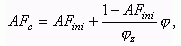where: fz is a combustion duration, CA.

Features of a designed procedure are:

• Step by step calculation of equilibrium composition of combustion products for eighteen species in the burnt gas zone./1/
• Kinetic calculation of thermal nitrogen oxides formation with chain Zeldovich mechanism /2/.

As for requirements of combustion of propellants in explosion engines defining the formation "thermal" NO is, in tendered model all calculations are yielded on the thermal mechanism.

Because in engine a  "thermal" NO are main, in simulation model all calculations are carried out with thermal mechanism.

The oxidizing of nitrogen be on the chain mechanism, basic reactions are:

O2   <=>  2O;                               (1)
N2 + O  <=>  NO + N;                       (2)
N + O2  <=>  NO + O.                       (3)

A main reaction is 3. Rate of this reaction depends on concentration of atomic oxygen.

The calculation of NO formation with equation of the chain mechanism is carried out for the zone of combustion, then the medial NO concentration over whole combustion chamber is determined. Volume concentration of NO in combustion products formed in a current calculation step is defined with equation: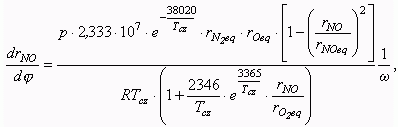where: p  is a cylinder pressure, Pa;
Tcz is a temperature in a burnt gas zone, K;
R  is a gas constant, J/(mole K);
w  is an angular crank velocity, 1/sec;
rNO eq, rN2 eq, rO eq, rO2 eq  are equilibrium concentrations of an oxide of nitrogen, molecular nitrogen, atomic and molecular oxygen, accordingly.

The equilibrium concentrations of 18 species are calculated on every time step. List of species includes:
O, O2, O3, H, H2, OH, H2O, C, CO, CO2, CH4, N, N2, NO, NO2, NH3, HNO3, HCN.
Overall system of equations includes: 14 equilibrium equations, 3 equations of material balance, and Dalton equation.

NO concentration in a cylinder:    rNO c = rNO  rbc,
where: rbc is a fraction of burnt gas in a cylinder.

NO concentration in a "dry" burnt gas of a cylinder:  rNO dry = rNO / (1 - rH2O),
where: rH2O is a volume fraction of water vapor in a combustion chamber.

Specific NO emission, g/kWh: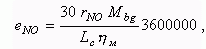where: Ìbg is a mass of burnt gas in a cylinder at the end of combustion, kmole;
Lc is working cycle work, kJ;
hì is a mechanical efficiency of engine.

Comparison of calculated NO emission with measured ones of truck diesel YaMZ-7512 working on 13 modes cycle is presented below.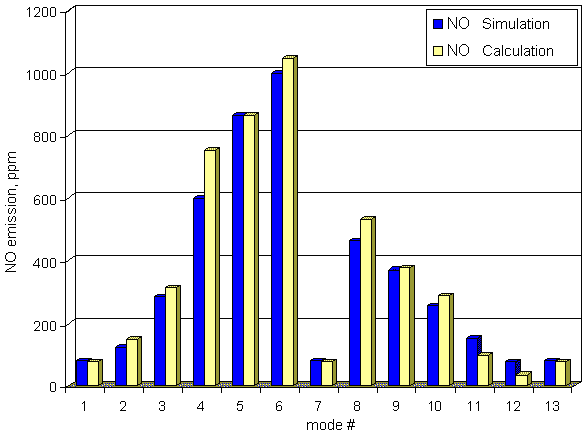Bibliography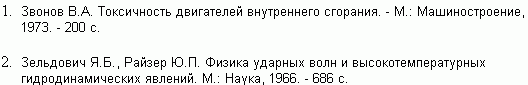To page "Calculation models"